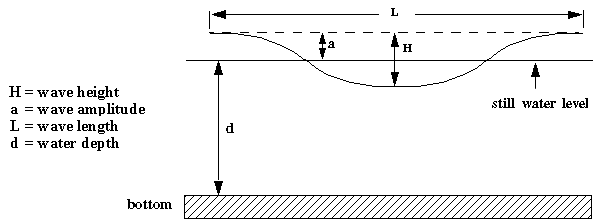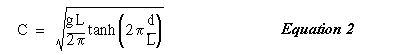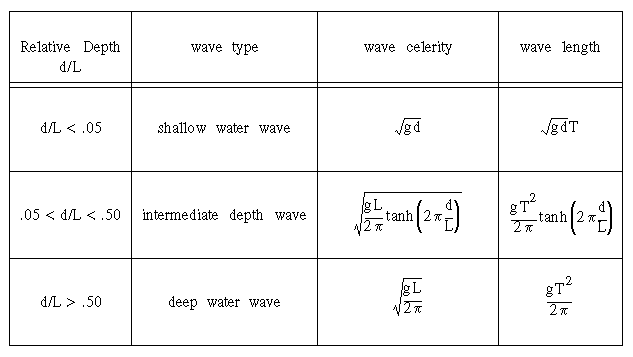# Water Wave Celerity

The three-dimensional behaviour of surface water waves as they interact with wind, bottom, obstructions, currents, and each other is very complex. Much insight into the general behaviour of waves can be obtained by first studying two-dimensional, monochromatic, progressive waves using the so-called small amplitude wave theory. The figure below defines the terms most commonly used in discussions of water waves:.As waves pass some fixed point, the time between consecutive crests is the wave period T. The speed of the wave, or its celerity, C, (as ocean engineers refer to it), is the distance travelled by a crest per unit time, or

`C = L/T                                            Equation 1`where g is the gravitational acceleration.